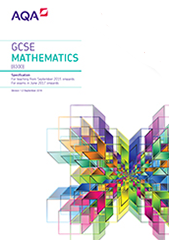# 3.4 Geometry and measures

## 3.4.1 Properties and constructions

### G1

Basic foundation content

Higher content only

use conventional terms and notations: points, lines, vertices, edges, planes, parallel lines, perpendicular lines, right angles, polygons, regular polygons and polygons with reflection and/or rotation symmetries

use the standard conventions for labelling and referring to the sides and angles of triangles

draw diagrams from written description

### G2

Basic foundation content

Higher content only

use the standard ruler and compass constructions (perpendicular bisector of a line segment, constructing a perpendicular to a given line from/at a given point, bisecting a given angle)

use these to construct given figures and solve loci problems

know that the perpendicular distance from a point to a line is the shortest distance to the line

Notes: including constructing an angle of 60°.

### G3

Basic foundation content

Higher content only

apply the properties of angles at a point, angles at a point on a straight line, vertically opposite angles

understand and use alternate and corresponding angles on parallel lines

derive and use the sum of angles in a triangle (eg to deduce and use the angle sum in any polygon, and to derive properties of regular polygons)

Notes: colloquial terms such as Z angles are not acceptable and should not be used.

### G4

Basic foundation content

Higher content only

derive and apply the properties and definitions of: special types of quadrilaterals, including square, rectangle, parallelogram, trapezium, kite and rhombus

and triangles and other plane figures using appropriate language

Notes: including knowing names and properties of isosceles, equilateral, scalene, right-angled, acute-angled, obtuse-angled triangles. Including knowing names and using the polygons: pentagon, hexagon, octagon and decagon.

### G5

Basic foundation content

Higher content only

use the basic congruence criteria for triangles (SSS, SAS, ASA, RHS)

### G6

Basic foundation content

Higher content only

apply angle facts, triangle congruence, similarity and properties of quadrilaterals to conjecture and derive results about angles and sides, including Pythagoras’ theorem and the fact that the base angles of an isosceles triangle are equal, and use known results to obtain simple proofs

### G7

Basic foundation content

Higher content only

identify, describe and construct congruent and similar shapes, including on coordinate axes, by considering rotation, reflection, translation and enlargement

including fractional scale factors

including negative scale factors

### G8

Basic foundation content

Higher content only

describe the changes and invariance achieved by combinations of rotations, reflections and translations

### G9

Basic foundation content

Higher content only

identify and apply circle definitions and properties, including: centre, radius, chord, diameter, circumference

including: tangent, arc, sector and segment

### G10

Basic foundation content

Higher content only

apply and prove the standard circle theorems concerning angles, radii, tangents and chords, and use them to prove related results

Notes: including angle subtended by an arc at the centre is equal to twice the angle subtended at any point on the circumference, angle subtended at the circumference by a semicircle is 90°, angles in the same segment are equal, opposite angles in a cyclic quadrilateral sum to 180°, tangent at any point on a circle is perpendicular to the radius at that point, tangents from an external point are equal in length, the perpendicular from the centre to a chord bisects the chord, alternate segment theorem.

### G11

Basic foundation content

Higher content only

solve geometrical problems on coordinate axes

### G12

Basic foundation content

Higher content only

identify properties of the faces, surfaces, edges and vertices of: cubes, cuboids, prisms, cylinders, pyramids, cones and spheres

### G13

Basic foundation content

Higher content only

interpret plans and elevations of 3D shapes

construct and interpret plans and elevations of 3D shapes

## 3.4.2 Mensuration and calculation

### G14

Basic foundation content

Higher content only

use standard units of measure and related concepts (length, area, volume/capacity, mass, time, money etc.)

### G15

Basic foundation content

Higher content only

measure line segments and angles in geometric figures, including interpreting maps and scale drawings and use of bearings

Notes: including the eight compass point bearings and three-figure bearings.

### G16

Basic foundation content

Higher content only

know and apply formulae to calculate: area of triangles, parallelograms, trapezia;

volume of cuboids and other right prisms (including cylinders)

### G17

Basic foundation content

Higher content only

know the formulae: circumference of a circle =

area of a circle =

calculate perimeters of 2D shapes, including circles

areas of circles and composite shapes

surface area and volume of spheres, pyramids, cones and composite solids

Notes: including frustums.

### G18

Basic foundation content

Higher content only

calculate arc lengths, angles and areas of sectors of circles

### G19

Basic foundation content

Higher content only

apply the concepts of congruence and similarity, including the relationships between lengths in similar figures

including the relationships between lengths, areas and volumes in similar figures

### G20

Basic foundation content

Higher content only

know the formulae for: Pythagoras’ theorem, and the trigonometric ratios, , and

apply them to find angles and lengths in right-angled triangles in two dimensional figures

apply them to find angles and lengths in right-angled triangles and, where possible, general triangles in two and three dimensional figures

### G21

Basic foundation content

Higher content only

know the exact values of and for = 0°, 30°, 45° , 60° and 90°

know the exact value of for = 0°, 30°, 45° , 60°

### G22

Basic foundation content

Higher content only

know and apply the sine rule,

and cosine rule,

to find unknown lengths and angles

### G23

Basic foundation content

Higher content only

know and apply

to calculate the area, sides or angles of any triangle

## 3.4.3 Vectors

### G24

Basic foundation content

Higher content only

describe translations as 2D vectors

### G25

Basic foundation content